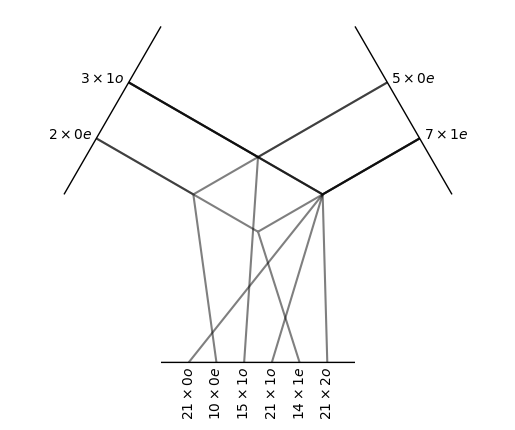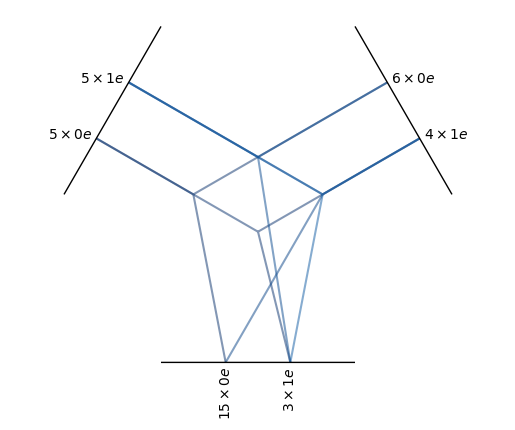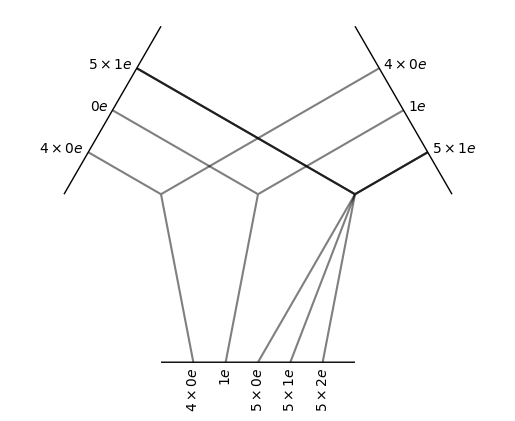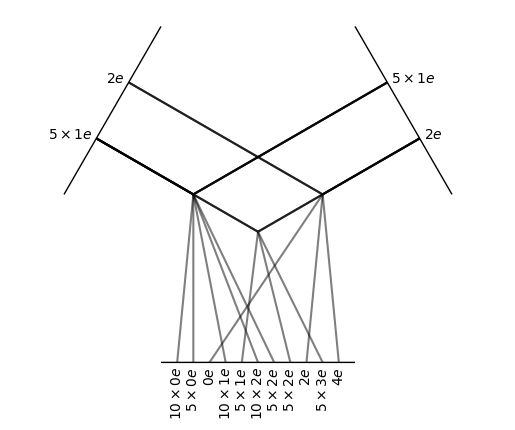# Tensor Product

All tensor products — denoted $$\otimes$$ — share two key characteristics:

1. The tensor product is bilinear: $$(\alpha x_1 + x_2) \otimes y = \alpha x_1 \otimes y + x_2 \otimes y$$ and $$x \otimes (\alpha y_1 + y_2) = \alpha x \otimes y_1 + x \otimes y_2$$

2. The tensor product is equivariant: $$(D x) \otimes (D y) = D (x \otimes y)$$ where $$D$$ is the representation of some symmetry operation from $$E(3)$$ (sorry for the very loose notation)

The class e3nn.o3.TensorProduct implements all possible tensor products between finite direct sums of irreducible representations (e3nn.o3.Irreps). While e3nn.o3.TensorProduct provides maximum flexibility, a number of sublcasses provide various typical special cases of the tensor product:

tp = o3.FullTensorProduct(
irreps_in1='2x0e + 3x1o',
irreps_in2='5x0e + 7x1e'
)
print(tp)
tp.visualize();

FullTensorProduct(2x0e+3x1o x 5x0e+7x1e -> 21x0o+10x0e+36x1o+14x1e+21x2o | 102 paths | 0 weights)The full tensor product is the “natural” one. Every possible output — each output irrep for every pair of input irreps — is created and returned independently. The outputs are not mixed with each other. Note how the multiplicities of the outputs are the product of the multiplicities of the respective inputs.

tp = o3.FullyConnectedTensorProduct(
irreps_in1='5x0e + 5x1e',
irreps_in2='6x0e + 4x1e',
irreps_out='15x0e + 3x1e'
)
print(tp)
tp.visualize();

FullyConnectedTensorProduct(5x0e+5x1e x 6x0e+4x1e -> 15x0e+3x1e | 960 paths | 960 weights)In a fully connected tensor product, all paths that lead to any of the irreps specified in irreps_out are created. Unlike e3nn.o3.FullTensorProduct, each output is a learned weighted sum of compatible paths. This allows e3nn.o3.FullyConnectedTensorProduct to produce outputs with any multiplicity; note that the example above has $$5 \times 6 + 5 \times 4 = 50$$ ways of creating scalars (0e), but the specified irreps_out has only 15 scalars, each of which is a learned weighted combination of those 50 possible scalars. The blue color in the visualization indicates that the path has these learnable weights.

All possible output irreps do not need to be included in irreps_out of a e3nn.o3.FullyConnectedTensorProduct: o3.FullyConnectedTensorProduct(irreps_in1='5x1o', irreps_in2='3x1o', irreps_out='20x0e') will only compute inner products between its inputs, since 1e, the output irrep of a vector cross product, is not present in irreps_out. Note also in this example that there are 20 output scalars, even though the given inputs can produce only 15 unique scalars — this is again allowed because each output is a learned linear combination of those 15 scalars, placing no restrictions on how many or how few outputs can be requested.

tp = o3.ElementwiseTensorProduct(
irreps_in1='5x0e + 5x1e',
irreps_in2='4x0e + 6x1e'
)
print(tp)
tp.visualize();

ElementwiseTensorProduct(5x0e+5x1e x 4x0e+6x1e -> 4x0e+1x1e+5x0e+5x1e+5x2e | 20 paths | 0 weights)In the elementwise tensor product, the irreps are multiplied one-by-one. Note in the visualization how the inputs have been split and that the multiplicities of the outputs match with the multiplicities of the input.

tp = o3.TensorSquare("5x1e + 2e")
print(tp)
tp.visualize();

TensorSquare(5x1e+1x2e -> 16x0e+15x1e+21x2e+5x3e+1x4e | 58 paths | 0 weights)The tensor square operation only computes the non-zero entries of a tensor times itself. It also applies different normalization rules taking into account that a tensor time itself is statistically different from the product of two independent tensors.

class e3nn.o3.TensorProduct(irreps_in1: Irreps, irreps_in2: Irreps, irreps_out: Irreps, instructions: , in1_var: Optional[Union[List[float], Tensor]] = None, in2_var: Optional[Union[List[float], Tensor]] = None, out_var: Optional[Union[List[float], Tensor]] = None, irrep_normalization: = None, path_normalization: = None, internal_weights: = None, shared_weights: = None, compile_left_right: bool = True, compile_right: bool = False, normalization=None, _specialized_code: = None, _optimize_einsums: = None)[source]

Bases: CodeGenMixin, Module

Tensor product with parametrized paths.

Parameters
• irreps_in1 (e3nn.o3.Irreps) – Irreps for the first input.

• irreps_in2 (e3nn.o3.Irreps) – Irreps for the second input.

• irreps_out (e3nn.o3.Irreps) – Irreps for the output.

• instructions (list of tuple) –

List of instructions (i_1, i_2, i_out, mode, train[, path_weight]).

Each instruction puts in1[i_1] $$\otimes$$ in2[i_2] into out[i_out].

• mode: str. Determines the way the multiplicities are treated, "uvw" is fully connected. Other valid

options are: 'uvw', 'uvu', 'uvv', 'uuw', 'uuu', and 'uvuv'. * train: bool. True if this path should have learnable weights, otherwise False. * path_weight: float. A fixed multiplicative weight to apply to the output of this path. Defaults to 1. Note that setting path_weight breaks the normalization derived from in1_var/in2_var/out_var.

• in1_var (list of float, Tensor, or None) – Variance for each irrep in irreps_in1. If None, all default to 1.0.

• in2_var (list of float, Tensor, or None) – Variance for each irrep in irreps_in2. If None, all default to 1.0.

• out_var (list of float, Tensor, or None) – Variance for each irrep in irreps_out. If None, all default to 1.0.

• irrep_normalization ({'component', 'norm'}) –

The assumed normalization of the input and output representations. If it is set to “norm”:

$\| x \| = \| y \| = 1 \Longrightarrow \| x \otimes y \| = 1$

• path_normalization ({'element', 'path'}) – If set to element, each output is normalized by the total number of elements (independently of their paths). If it is set to path, each path is normalized by the total number of elements in the path, then each output is normalized by the number of paths.

• internal_weights (bool) – whether the e3nn.o3.TensorProduct contains its learnable weights as a parameter

• shared_weights (bool) –

whether the learnable weights are shared among the input’s extra dimensions

where here $$i$$ denotes a batch-like index. shared_weights cannot be False if internal_weights is True.

• compile_left_right (bool) – whether to compile the forward function, true by default

• compile_right (bool) – whether to compile the .right function, false by default

Examples

Create a module that computes elementwise the cross-product of 16 vectors with 16 vectors $$z_u = x_u \wedge y_u$$

>>> module = TensorProduct(
...     "16x1o", "16x1o", "16x1e",
...     [
...         (0, 0, 0, "uuu", False)
...     ]
... )


Now mix all 16 vectors with all 16 vectors to makes 16 pseudo-vectors $$z_w = \sum_{u,v} w_{uvw} x_u \wedge y_v$$

>>> module = TensorProduct(
...     [(16, (1, -1))],
...     [(16, (1, -1))],
...     [(16, (1,  1))],
...     [
...         (0, 0, 0, "uvw", True)
...     ]
... )


With custom input variance and custom path weights:

>>> module = TensorProduct(
...     "8x0o + 8x1o",
...     "16x1o",
...     "16x1e",
...     [
...         (0, 0, 0, "uvw", True, 3),
...         (1, 0, 0, "uvw", True, 1),
...     ],
...     in2_var=[1/16]
... )


Example of a dot product:

>>> irreps = o3.Irreps("3x0e + 4x0o + 1e + 2o + 3o")
>>> module = TensorProduct(irreps, irreps, "0e", [
...     (i, i, 0, 'uuw', False)
...     for i, (mul, ir) in enumerate(irreps)
... ])


Implement $$z_u = x_u \otimes (\sum_v w_{uv} y_v)$$

>>> module = TensorProduct(
...     "8x0o + 7x1o + 3x2e",
...     "10x0e + 10x1e + 10x2e",
...     "8x0o + 7x1o + 3x2e",
...     [
...         # paths for the l=0:
...         (0, 0, 0, "uvu", True),  # 0x0->0
...         # paths for the l=1:
...         (1, 0, 1, "uvu", True),  # 1x0->1
...         (1, 1, 1, "uvu", True),  # 1x1->1
...         (1, 2, 1, "uvu", True),  # 1x2->1
...         # paths for the l=2:
...         (2, 0, 2, "uvu", True),  # 2x0->2
...         (2, 1, 2, "uvu", True),  # 2x1->2
...         (2, 2, 2, "uvu", True),  # 2x2->2
...     ]
... )


Tensor Product using the xavier uniform initialization:

>>> irreps_1 = o3.Irreps("5x0e + 10x1o + 1x2e")
>>> irreps_2 = o3.Irreps("5x0e + 10x1o + 1x2e")
>>> irreps_out = o3.Irreps("5x0e + 10x1o + 1x2e")
>>> # create a Fully Connected Tensor Product
>>> module = o3.TensorProduct(
...     irreps_1,
...     irreps_2,
...     irreps_out,
...     [
...         (i_1, i_2, i_out, "uvw", True, mul_1 * mul_2)
...         for i_1, (mul_1, ir_1) in enumerate(irreps_1)
...         for i_2, (mul_2, ir_2) in enumerate(irreps_2)
...         for i_out, (mul_out, ir_out) in enumerate(irreps_out)
...         if ir_out in ir_1 * ir_2
...     ]
... )
...     for weight in module.weight_views():
...         mul_1, mul_2, mul_out = weight.shape
...         # formula from torch.nn.init.xavier_uniform_
...         a = (6 / (mul_1 * mul_2 + mul_out))**0.5
...         new_weight = torch.empty_like(weight)
...         new_weight.uniform_(-a, a)
...         weight[:] = new_weight
tensor(...)
>>> n = 1_000
>>> vars = module(irreps_1.randn(n, -1), irreps_2.randn(n, -1)).var(0)
>>> assert vars.min() > 1 / 3
>>> assert vars.max() < 3


Methods:

 forward(x, y[, weight]) Evaluate $$w x \otimes y$$. right(y[, weight]) Partially evaluate $$w x \otimes y$$. visualize([weight, plot_weight, ...]) Visualize the connectivity of this e3nn.o3.TensorProduct weight_view_for_instruction(instruction[, ...]) View of weights corresponding to instruction. weight_views([weight, yield_instruction]) Iterator over weight views for each weighted instruction.
forward(x, y, weight: = None)[source]

Evaluate $$w x \otimes y$$.

Parameters
• x (torch.Tensor) – tensor of shape (..., irreps_in1.dim)

• y (torch.Tensor) – tensor of shape (..., irreps_in2.dim)

• weight (torch.Tensor or list of torch.Tensor, optional) – required if internal_weights is False tensor of shape (self.weight_numel,) if shared_weights is True tensor of shape (..., self.weight_numel) if shared_weights is False or list of tensors of shapes weight_shape / (...) + weight_shape. Use self.instructions to know what are the weights used for.

Returns

tensor of shape (..., irreps_out.dim)

Return type

torch.Tensor

right(y, weight: = None)[source]

Partially evaluate $$w x \otimes y$$.

It returns an operator in the form of a tensor that can act on an arbitrary $$x$$.

For example, if the tensor product above is expressed as

$w_{ijk} x_i y_j \rightarrow z_k$

then the right method returns a tensor $$b_{ik}$$ such that

$w_{ijk} y_j \rightarrow b_{ik} x_i b_{ik} \rightarrow z_k$

The result of this method can be applied with a tensor contraction:

torch.einsum("...ik,...i->...k", right, input)

Parameters
• y (torch.Tensor) – tensor of shape (..., irreps_in2.dim)

• weight (torch.Tensor or list of torch.Tensor, optional) – required if internal_weights is False tensor of shape (self.weight_numel,) if shared_weights is True tensor of shape (..., self.weight_numel) if shared_weights is False or list of tensors of shapes weight_shape / (...) + weight_shape. Use self.instructions to know what are the weights used for.

Returns

tensor of shape (..., irreps_in1.dim, irreps_out.dim)

Return type

torch.Tensor

visualize(weight: = None, plot_weight: bool = True, aspect_ratio=1, ax=None)[source]

Visualize the connectivity of this e3nn.o3.TensorProduct

Parameters
• weight (torch.Tensor, optional) – like weight argument to forward()

• plot_weight (bool, default True) – Whether to color paths by the sum of their weights.

• ax (matplotlib.Axes, default None) – The axes to plot on. If None, a new figure will be created.

Returns

The figure and axes on which the plot was drawn.

Return type

(fig, ax)

weight_view_for_instruction(instruction: int, weight: = None) [source]

View of weights corresponding to instruction.

Parameters
• instruction (int) – The index of the instruction to get a view on the weights for. self.instructions[instruction].has_weight must be True.

• weight (torch.Tensor, optional) – like weight argument to forward()

Returns

A view on weight or this object’s internal weights for the weights corresponding to the instruction th instruction.

Return type

torch.Tensor

weight_views(weight: = None, yield_instruction: bool = False)[source]

Iterator over weight views for each weighted instruction.

Parameters
• weight (torch.Tensor, optional) – like weight argument to forward()

• yield_instruction (bool, default False) – Whether to also yield the corresponding instruction.

Yields
• If yield_instruction is True, yields (instruction_index, instruction, weight_view).

• Otherwise, yields weight_view.

class e3nn.o3.FullyConnectedTensorProduct(irreps_in1, irreps_in2, irreps_out, irrep_normalization: = None, path_normalization: = None, **kwargs)[source]

Bases: TensorProduct

Fully-connected weighted tensor product

All the possible path allowed by $$|l_1 - l_2| \leq l_{out} \leq l_1 + l_2$$ are made. The output is a sum on different paths:

$z_w = \sum_{u,v} w_{uvw} x_u \otimes y_v + \cdots \text{other paths}$

where $$u,v,w$$ are the indices of the multiplicities.

Parameters
class e3nn.o3.FullTensorProduct(irreps_in1: Irreps, irreps_in2: Irreps, filter_ir_out: = None, irrep_normalization: = None, **kwargs)[source]

Bases: TensorProduct

Full tensor product between two irreps.

$z_{uv} = x_u \otimes y_v$

where $$u$$ and $$v$$ run over the irreps. Note that there are no weights. The output representation is determined by the two input representations.

Parameters
class e3nn.o3.ElementwiseTensorProduct(irreps_in1, irreps_in2, filter_ir_out=None, irrep_normalization: = None, **kwargs)[source]

Bases: TensorProduct

Elementwise connected tensor product.

$z_u = x_u \otimes y_u$

where $$u$$ runs over the irreps. Note that there are no weights. The output representation is determined by the two input representations.

Parameters

Examples

Elementwise scalar product

>>> ElementwiseTensorProduct("5x1o + 5x1e", "10x1e", ["0e", "0o"])
ElementwiseTensorProduct(5x1o+5x1e x 10x1e -> 5x0o+5x0e | 10 paths | 0 weights)

class e3nn.o3.TensorSquare(irreps_in: Irreps, irreps_out: = None, filter_ir_out: = None, irrep_normalization: = None, **kwargs)[source]

Bases: TensorProduct

Compute the square tensor product of a tensor and reduce it in irreps

If irreps_out is given, this operation is fully connected. If irreps_out is not given, the operation has no parameter and is like full tensor product.

Parameters

Methods:

 forward(x[, weight]) Evaluate $$w x \otimes y$$.
forward(x, weight: = None)[source]

Evaluate $$w x \otimes y$$.

Parameters
• x (torch.Tensor) – tensor of shape (..., irreps_in1.dim)

• y (torch.Tensor) – tensor of shape (..., irreps_in2.dim)

• weight (torch.Tensor or list of torch.Tensor, optional) – required if internal_weights is False tensor of shape (self.weight_numel,) if shared_weights is True tensor of shape (..., self.weight_numel) if shared_weights is False or list of tensors of shapes weight_shape / (...) + weight_shape. Use self.instructions to know what are the weights used for.

Returns

tensor of shape (..., irreps_out.dim)

Return type

torch.Tensor